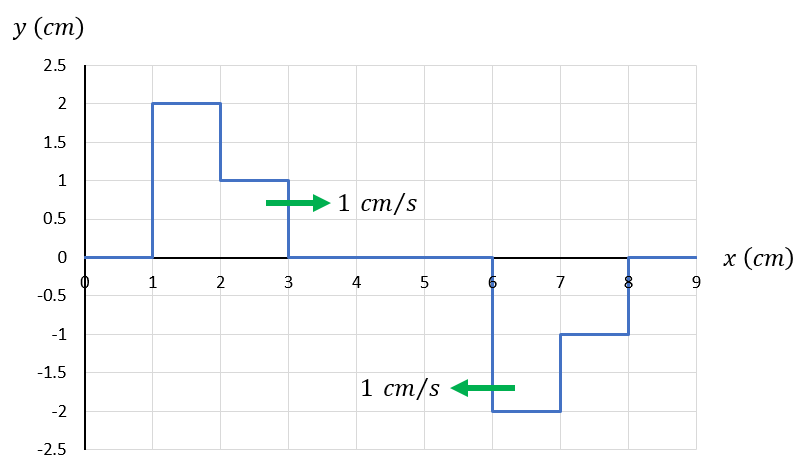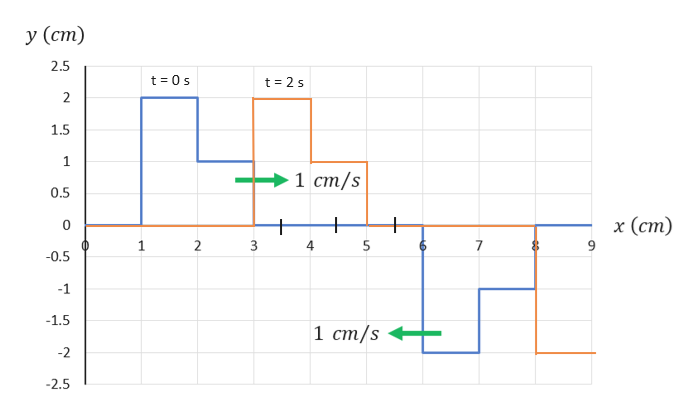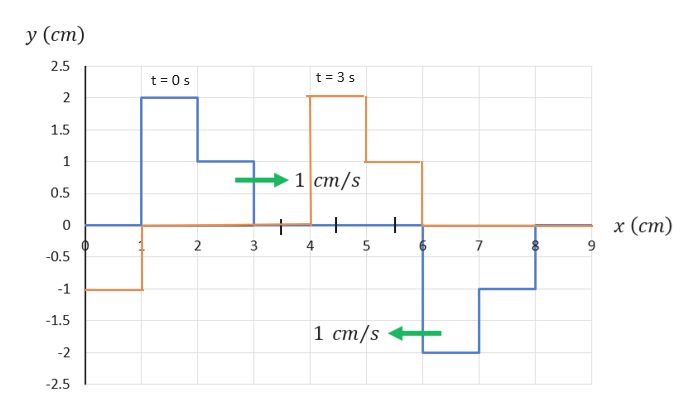# у (ст)2.521.511 ст/s0.5х (ст)0123457-0.5-1-1.51 ст/s-2-2.5— о-

Question
10 views

Two wave pulses travel on a string. The picture shows the displacement of the string y as a function of x at t = 0. The velocities of the wave pulses are shown.

1. At t = 2 s, what is the displacement of the string at the following values of x?

At x = 3.5 cm, y =  ______ cm
At x = 4.5 cm, y =  ______ cm
At x = 5.5 cm, y =  ______ cm

2. At t = 3 s, what is the displacement of the string at the following values of x?

At x = 3.5 cm, y =  ______ cm
At x = 4.5 cm, y =  ______ cm
At x = 5.5 cm, y =  ______ cmhelp_outlineImage Transcriptioncloseу (ст) 2.5 2 1.5 1 1 ст/s 0.5 х (ст) 0 1 2 3 4 5 7 -0.5 -1 -1.5 1 ст/s -2 -2.5 — о- fullscreen
check_circle

Step 1

Calculating the y direction displacement of the string at given values of x at t = 2 s:

A graph has been constructed with orange color on the same given graph, which represent the position of the string at t = 2 s. As the velocity of pulse is 1 cm/s in x direction, so the wave will shift to 2 cm towards x-axis after 2 seconds. As shown in figure:

Hence, from diagram, we can observe that the displacement of the wave:

At x = 3.5 cm is y = 2 cm

At x = 4.5 cm is y = 1 cm

At x = 5.5 cm is y = 0 cmhelp_outlineImage Transcriptioncloseу (ст) 2.5 t 0s t 2 s 2 1.5 1 1 ст/s 0.5 x (ст) 0 1 2 4 5 7 -0.5 -1 -1.5 1 ст/s -2 2.5 st fullscreen
Step 2

Calculating the y direction displacement of the string at given values of x at t = 3 s:

A graph has been constructed with orange color on the same given graph, which represent the position of the string at t = 3 s. As the velocity of pulse is 1 cm/s in x direction, ...help_outlineImage Transcriptioncloseу (ст) 2.5 t 3 s t 0 s 2 1.5 1 1 ст/s 0.5 x (ст) 0 2 5 7 -0.5 -1 -1.5 1 ст/s -2 2.5 st fullscreen

### Want to see the full answer?

See Solution

#### Want to see this answer and more?

Solutions are written by subject experts who are available 24/7. Questions are typically answered within 1 hour.*

See Solution
*Response times may vary by subject and question.
Tagged in

### Other